M362K Second Midterm Exam Solutions
March 7, 2002

Problem 1. Political committees

13 Democrats, 12 Republicans and 8 Independents are sitting in a room. 8 of these people will be selected to serve on a special committee.

a) How many different possibilities are there for the committee membership?

You must choose 8 of the 33 people, so there are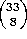possibilities.

b) What is the probability that exactly 5 of the committee members will be Democrats?

This is hypergeometric: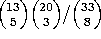c) What is the probability that the committee will consist of 4 Democrats, 3 Republicans and one independent?

The number of ways to do this is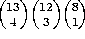, so the probability is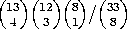.

Problem 2. Number theory

a) How many solutions exist to the equation x+y+z = 15, where x, y, and z have to be non-negative integers? Simplify your answer as much as possible. [Note: the solution x=12, y=2, z=1 is not the same as x=1, y=2, z=12]

This is the same as the ``give \$20 to the mailman, babysitter and garbage man'' problem. We are choosing 15 out of 3 items, repetition OK, order unimportant, and so can do it in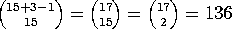ways.

b) How many solutions exist to the equation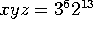, where x, y and z have to be positive integers?

This is really two copies of part (a). Write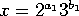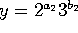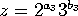. Then we must have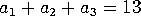and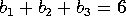. There are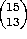ways to solve the first equation and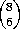ways to solve the second. The answer is then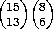.

Problem 3. Spring Break Drinking

On South Padre Island over spring break, 25 underage students try to buy beer with fake IDs. Each has a 10% chance of getting caught, independent of the others.

a) What is the probability that exactly 4 of the students get caught?

This is binomial, with p=0.1 and n=25. The answer is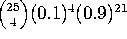.

b) What is the probability that 3 or more students get caught?

It's easier to figure the probability that two or fewer get caught, and subtract from 1. The final answer is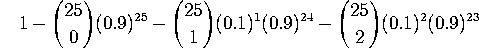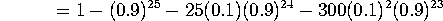0.46291

Problem 4. Grab bag

a) How many 9-letter license plates can be made by rearranging the letters of the phrase ``I HATE MATH''?

There are 2 A's, 2 H's, 2 T's one E, one I, and one M, so there are 9! / (2!2!2!1!1!1!) = 9!/8 ways.

b) 25 children submit science fair projects. The judges will award a 1st-place trophy, a 2nd place trophy, a 3rd place trophy, a 4th place trophy, a 5th place trophy, five identical ``honorable mention'' ribbons (for the 6th-10th best projects), and participation ribbons for the remaining children. In how many different ways can the judges distribute the prizes?

There are 25!/20! to distribute the trophies, then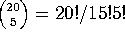ways to distribute the honorable mention ribbons, and then one way to distribute the participation ribbons, for a total of 25!/15!5! ways in all.